• Slides: 36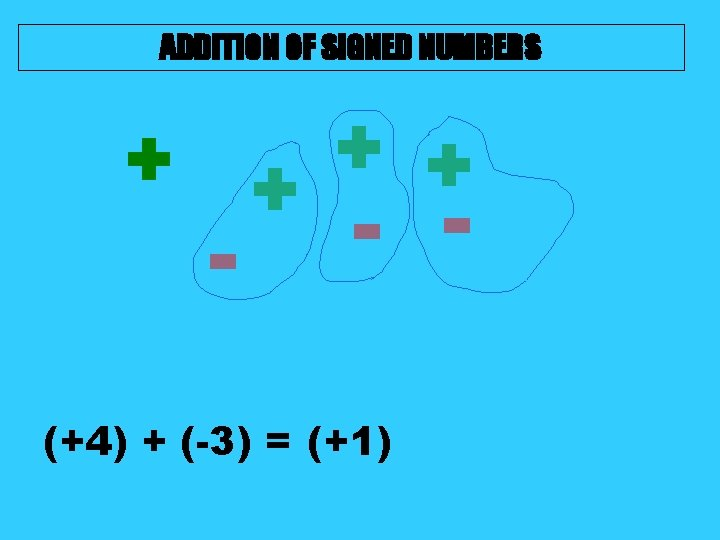ADDITION OF SIGNED NUMBERS + + - - (+4) + (-3) = (+1)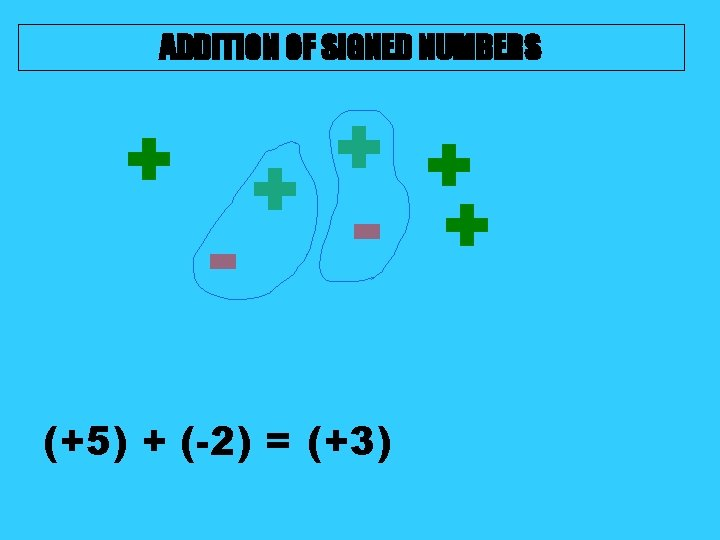ADDITION OF SIGNED NUMBERS + + - + - (+5) + (-2) = (+3)ADDITION OF SIGNED NUMBERS + - (+2) + (-6) = (-4) - -ADDITION OF SIGNED NUMBERS + + - (+3) + (-5) = (-2)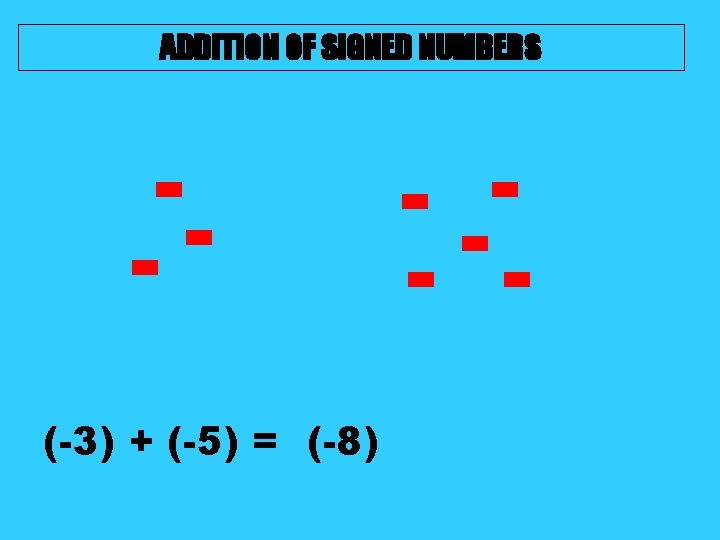ADDITION OF SIGNED NUMBERS - (-3) + (-5) = (-8) - - -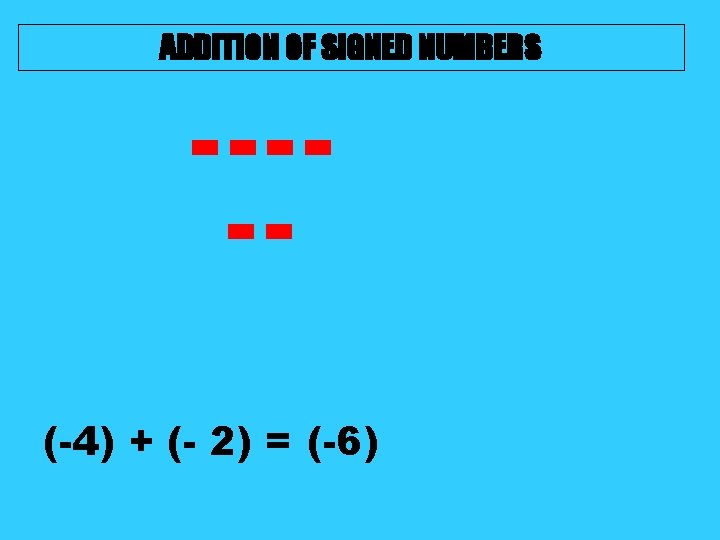ADDITION OF SIGNED NUMBERS ----(-4) + (- 2) = (-6)ADDITION OF SIGNED NUMBERS +++++++ (+5) + (+7) = (+12)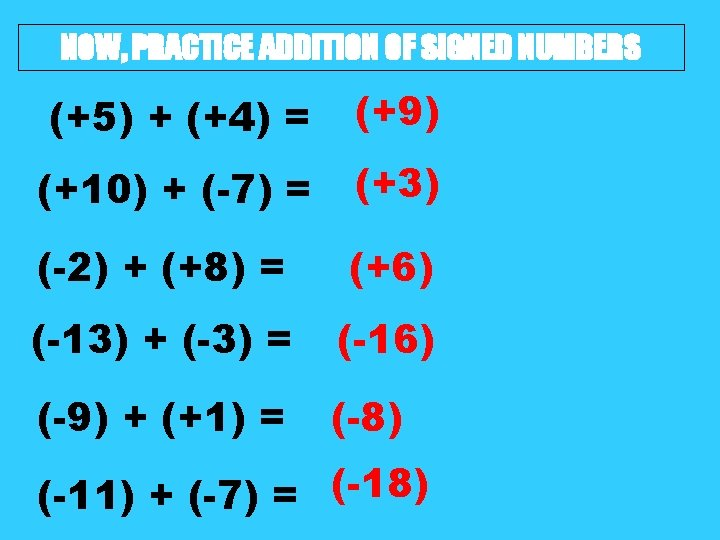NOW, PRACTICE ADDITION OF SIGNED NUMBERS (+5) + (+4) = (+9) (+10) + (-7) = (+3) (-2) + (+8) = (+6) (-13) + (-3) = (-16) (-9) + (+1) = (-8) (-11) + (-7) = (-18)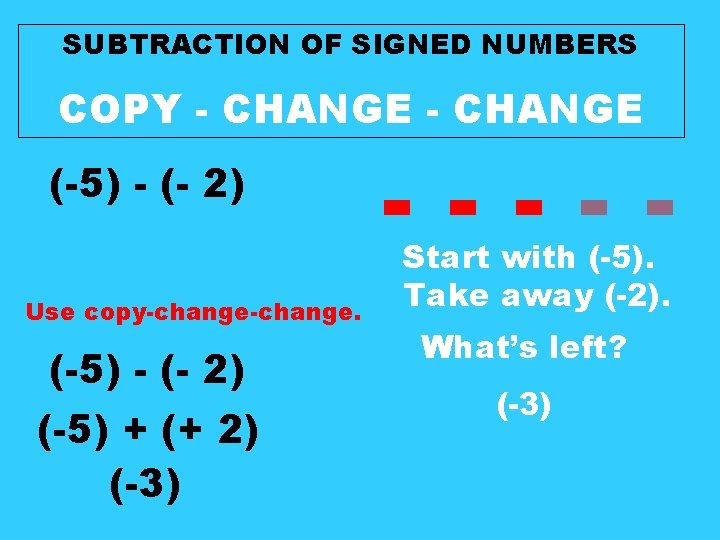SUBTRACTION OF SIGNED NUMBERS COPY - CHANGE (-5) - (- 2) Use copy-change. (-5) - (- 2) (-5) + (+ 2) (-3) - - Start with (-5). Take away (-2). What’s left? (-3)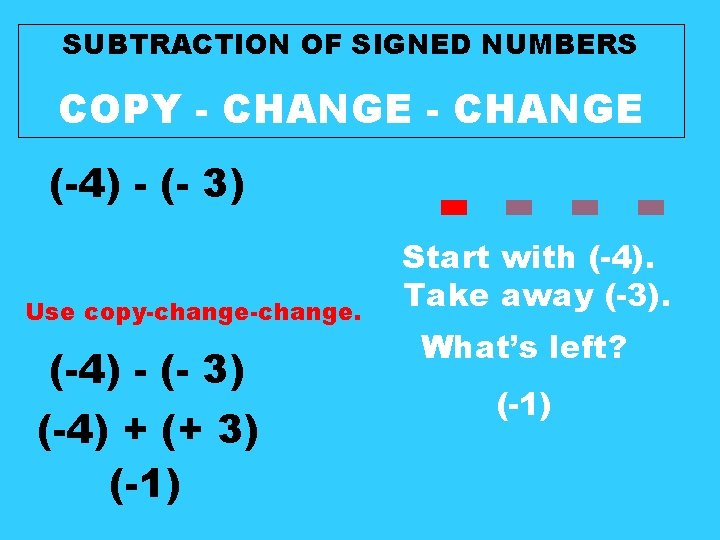SUBTRACTION OF SIGNED NUMBERS COPY - CHANGE (-4) - (- 3) Use copy-change. (-4) - (- 3) (-4) + (+ 3) (-1) - - Start with (-4). Take away (-3). What’s left? (-1)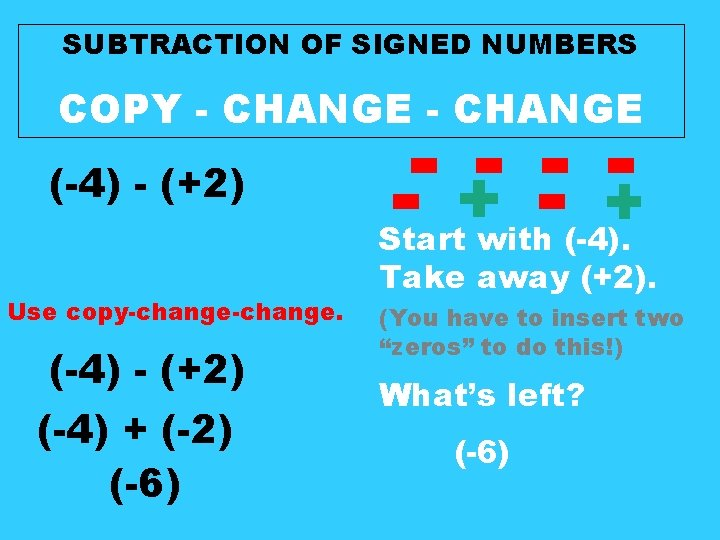SUBTRACTION OF SIGNED NUMBERS COPY - CHANGE (-4) - (+2) Use copy-change. (-4) - (+2) (-4) + (-2) (-6) - + Start with (-4). Take away (+2). (You have to insert two “zeros” to do this!) What’s left? (-6)NOW, PRACTICE SUBTRACTION OF SIGNED NUMBERS USE COPY - CHANGE (+11) - (-6) Use copy-change. (+11) + (+6) (+17)(-12) - (-2) Use copy-change. (-12) + (+2) (-10)(-8) - (+20) Use copy-change. (-8) + (-20) (-28)(+15) - (-4) Use copy-change. (+15) + (+4) (+19)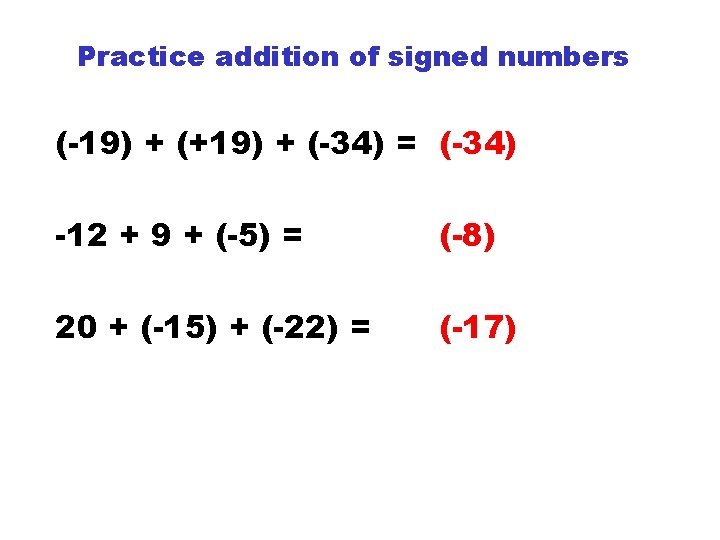Practice addition of signed numbers (-19) + (+19) + (-34) = (-34) -12 + 9 + (-5) = (-8) 20 + (-15) + (-22) = (-17)Practice subtraction of signed numbers (+5) – (+12 = (-7) 6 – (-16) = (+22) -11 – (-7) = (-4) -9 – (-10) = (+1)MULTIPLICATION OF SIGNED NUMBERS ---- Start with (-4). ---- Multiply it by 3. That means that you will have 3 groups of (-4). (3)(-4) = (-12)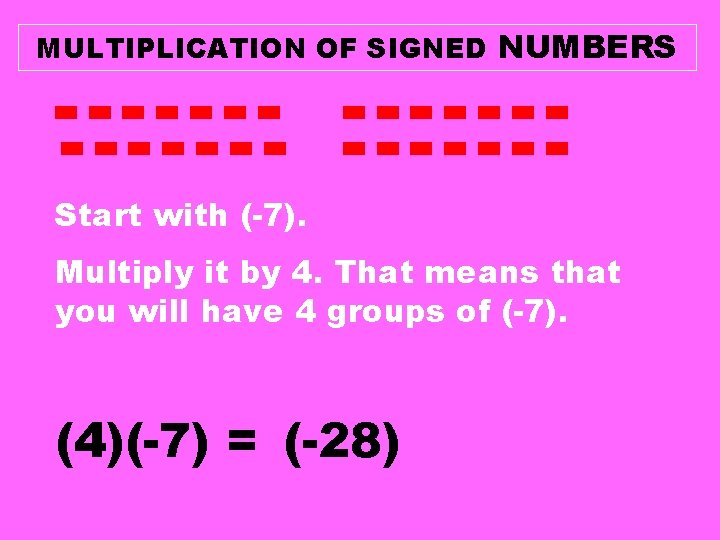MULTIPLICATION OF SIGNED NUMBERS -- -- -- -- -Start with (-7). Multiply it by 4. That means that you will have 4 groups of (-7). (4)(-7) = (-28)MULTIPLICATION OF SIGNED NUMBERS +++++ +++++ Start with (+5). Multiply it by 5. That means that you will have 5 groups of (+5). (5)(+5) = (+25)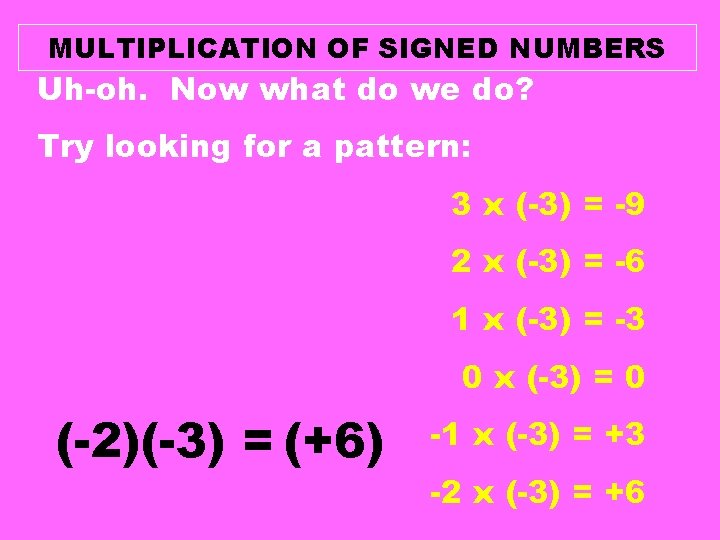MULTIPLICATION OF SIGNED NUMBERS Uh-oh. Now what do we do? Try looking for a pattern: 3 x (-3) = -9 2 x (-3) = -6 1 x (-3) = -3 0 x (-3) = 0 (-2)(-3) = (+6) -1 x (-3) = +3 -2 x (-3) = +6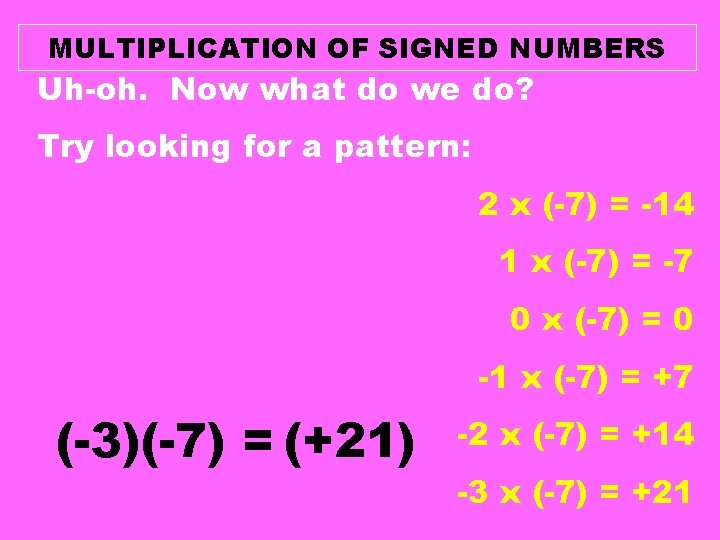MULTIPLICATION OF SIGNED NUMBERS Uh-oh. Now what do we do? Try looking for a pattern: 2 x (-7) = -14 1 x (-7) = -7 0 x (-7) = 0 -1 x (-7) = +7 (-3)(-7) = (+21) -2 x (-7) = +14 -3 x (-7) = +21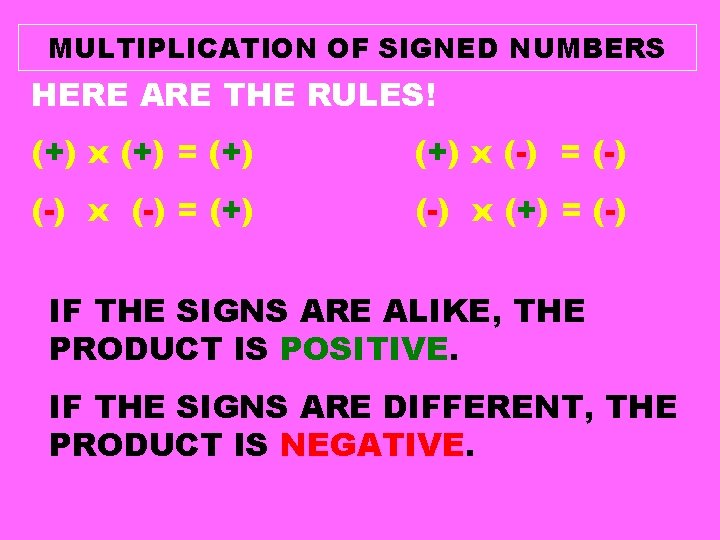MULTIPLICATION OF SIGNED NUMBERS HERE ARE THE RULES! (+) x (+) = (+) x (-) = (-) x (-) = (+) (-) x (+) = (-) IF THE SIGNS ARE ALIKE, THE PRODUCT IS POSITIVE. IF THE SIGNS ARE DIFFERENT, THE PRODUCT IS NEGATIVE.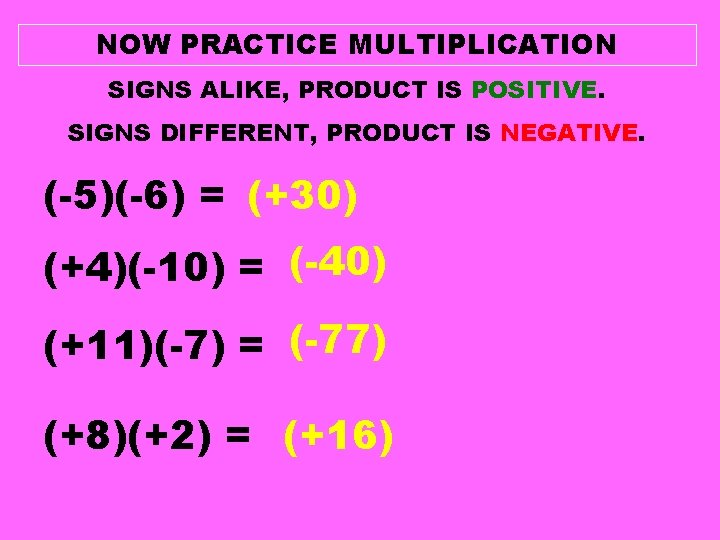NOW PRACTICE MULTIPLICATION SIGNS ALIKE, PRODUCT IS POSITIVE. SIGNS DIFFERENT, PRODUCT IS NEGATIVE. (-5)(-6) = (+30) (+4)(-10) = (-40) (+11)(-7) = (-77) (+8)(+2) = (+16)DIVISION OF SIGNED NUMBERS -------Start with (-15). Split it into 3 equal sections. (-15) (3) = (-5)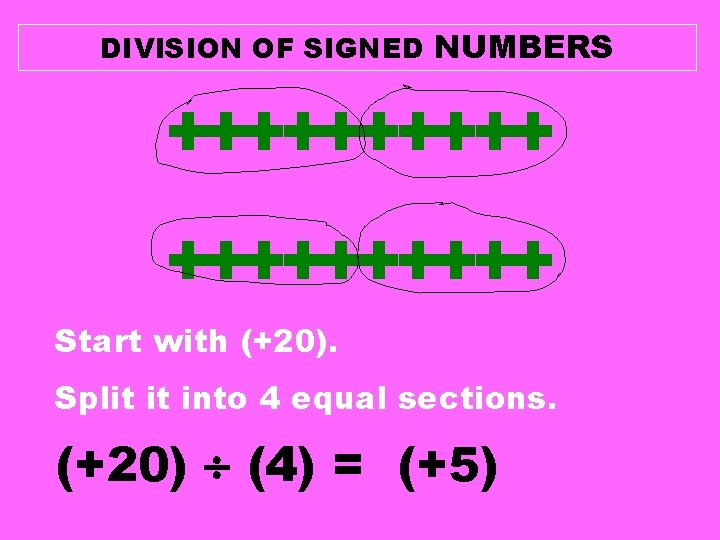DIVISION OF SIGNED NUMBERS ++++++++++ Start with (+20). Split it into 4 equal sections. (+20) (4) = (+5)DIVISION OF SIGNED NUMBERS HERE ARE THE RULES! (+) = (+) (-) = (-) = (+) (-) (+) = (-) IF THE SIGNS ARE ALIKE, THE QUOTIENT IS POSITIVE. IF THE SIGNS ARE DIFFERENT, THE QUOTIENT IS NEGATIVE.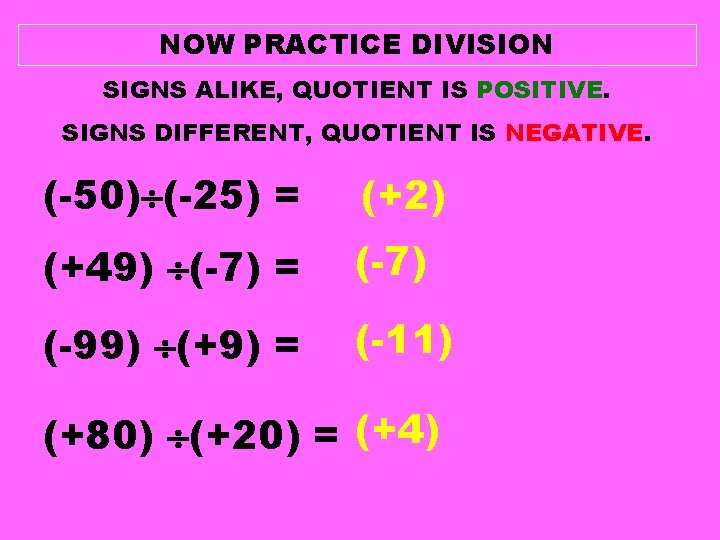NOW PRACTICE DIVISION SIGNS ALIKE, QUOTIENT IS POSITIVE. SIGNS DIFFERENT, QUOTIENT IS NEGATIVE. (-50) (-25) = (+2) (+49) (-7) = (-7) (-99) (+9) = (-11) (+80) (+20) = (+4)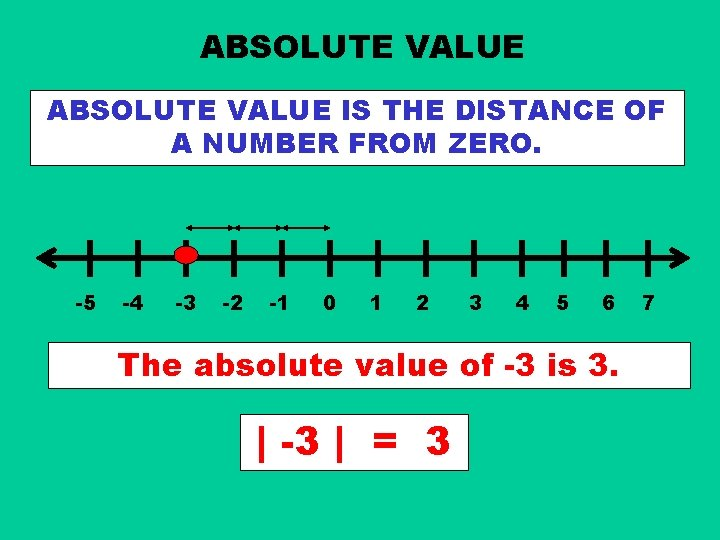ABSOLUTE VALUE IS THE DISTANCE OF A NUMBER FROM ZERO. -5 -4 -3 -2 -1 0 1 2 3 4 5 6 The absolute value of -3 is 3. | -3 | = 3 7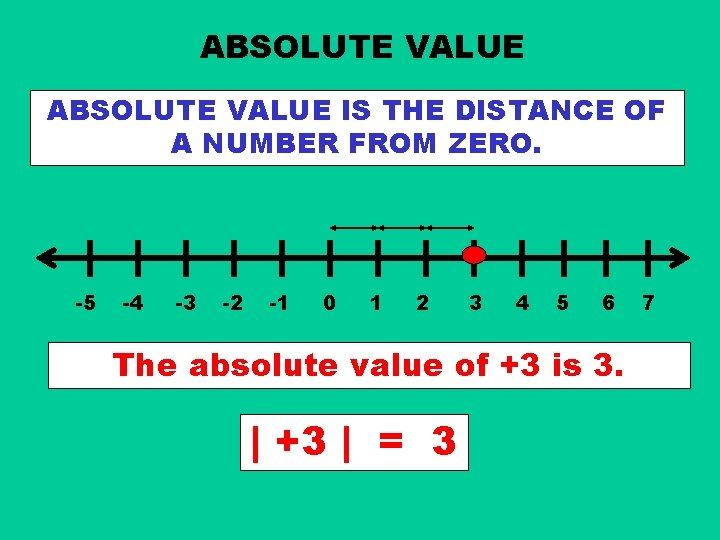ABSOLUTE VALUE IS THE DISTANCE OF A NUMBER FROM ZERO. -5 -4 -3 -2 -1 0 1 2 3 4 5 6 The absolute value of +3 is 3. | +3 | = 3 7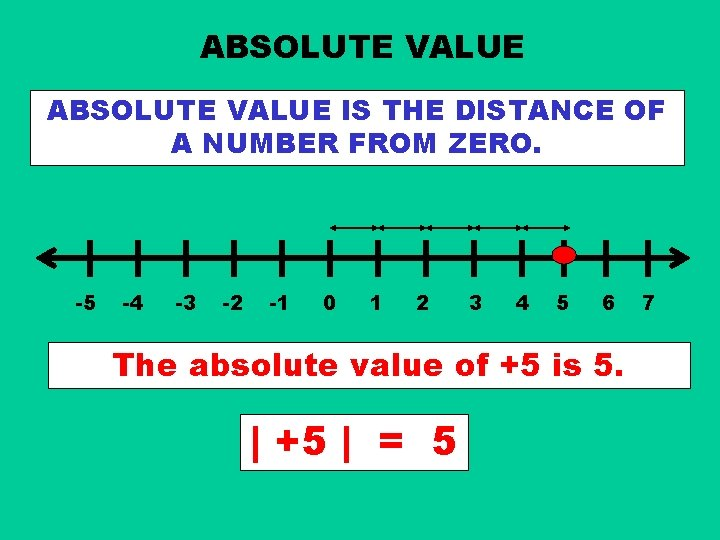ABSOLUTE VALUE IS THE DISTANCE OF A NUMBER FROM ZERO. -5 -4 -3 -2 -1 0 1 2 3 4 5 6 The absolute value of +5 is 5. | +5 | = 5 7ABSOLUTE VALUE IS THE DISTANCE OF A NUMBER FROM ZERO. -5 -4 -3 -2 -1 0 1 2 3 4 5 6 The absolute value of -5 is 5. | -5 | = 5 7ABSOLUTE VALUE IS THE DISTANCE OF A NUMBER FROM ZERO. -5 -4 -3 -2 -1 0 1 2 3 4 5 6 The absolute value of 0 is 0. |0| = 0 7ABSOLUTE VALUE DISTANCE IS ALWAYS A POSITIVE NUMBER!! -5 -4 -3 -2 -1 0 1 2 3 4 5 6 The absolute value of -4 is 4. | -4 | = 4 7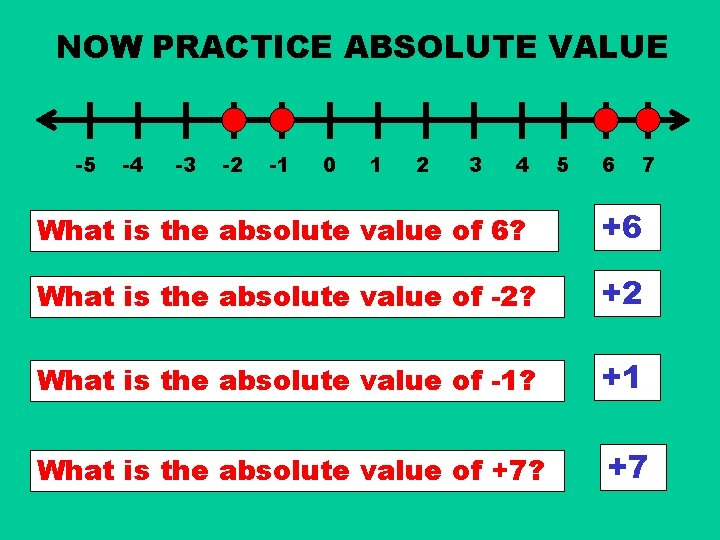NOW PRACTICE ABSOLUTE VALUE -5 -4 -3 -2 -1 0 1 2 3 4 5 6 7 What is the absolute value of 6? +6 What is the absolute value of -2? +2 What is the absolute value of -1? +1 What is the absolute value of +7? +7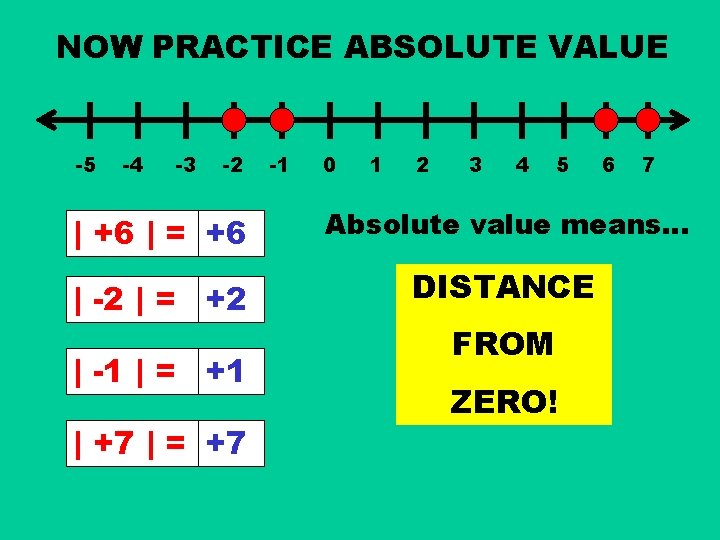NOW PRACTICE ABSOLUTE VALUE -5 -4 -3 -2 -1 0 1 2 3 4 5 6 7 | +6 | = +6 Absolute value means… | -2 | = +2 DISTANCE | -1 | = +1 | +7 | = +7 FROM ZERO!